NCERT Solutions: Visualising Solid Shapes- 2

# NCERT Solutions: Visualising Solid Shapes- 2 - Notes | Study Mathematics (Maths) Class 7 - Class 7

 1 Crore+ students have signed up on EduRev. Have you?

Exercise 15.3

Question 1:

What cross-sections do you get when you give a:
(i) vertical cut (ii) horizontal cut to the following solids?
(a) A brick
(b) A round apple
(c) A die
(d) A circular pipe
(e) An ice-cream cone.

 S.No. Name of article Figure Vertical cut Horizontal cut (a) A brick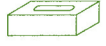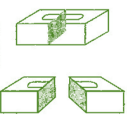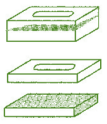(b) A round apple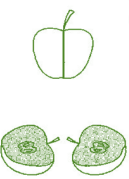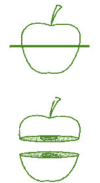(c) A die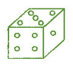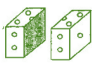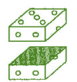(d) A circular pipe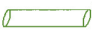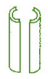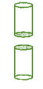(e) An ice-cream cone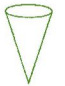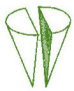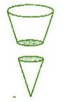Exercise 15.4

Question 1:

A bulb is kept burning just right above the following solids. Name the shape of the shadows obtained in each case. Attempt to give a rough sketch of the shadow.

(You may try to experiment first and then answer these questions).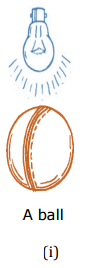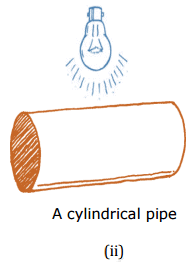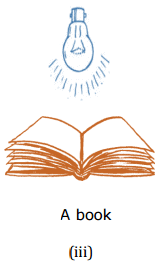S.No. Object Shadow Shape's name (i) A ball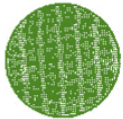Circle (ii) A cylindrical pipe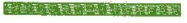Line (iii) A book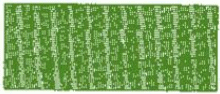Rectangle

Question 2:

Here are the shadows of some 3-D objects, when seen under the lamp of the overhead projector. Identify the solid (s) that match each shadow.

(There may be multiple answers for these!)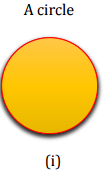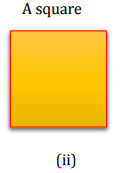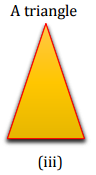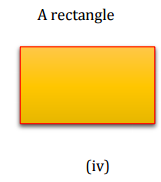S. No. Shadow Shape's Name 3-D objects (i)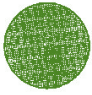Circle Chapatti, Football, Disc, Plate etc. (ii)Square Die, Square paper sheet, cubical magic box, Chalk box etc. (iii)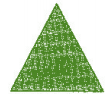Triangle Ice-cream cone, Birthday cap, etc. (iv)Rectangle Geometry box, Book, Table etc.

Question 3:

Examine if the following are true statements:

(i) The cube can cast a shadow in the shape of a rectangle.

(ii) The cube can cast a shadow in the shape of a hexagon.

(i) True

(ii) False

The document NCERT Solutions: Visualising Solid Shapes- 2 - Notes | Study Mathematics (Maths) Class 7 - Class 7 is a part of the Class 7 Course Mathematics (Maths) Class 7.
All you need of Class 7 at this link: Class 7

## Mathematics (Maths) Class 7

168 videos|276 docs|45 tests
 Use Code STAYHOME200 and get INR 200 additional OFF

## Mathematics (Maths) Class 7

168 videos|276 docs|45 tests

Track your progress, build streaks, highlight & save important lessons and more!

,

,

,

,

,

,

,

,

,

,

,

,

,

,

,

,

,

,

,

,

,

;